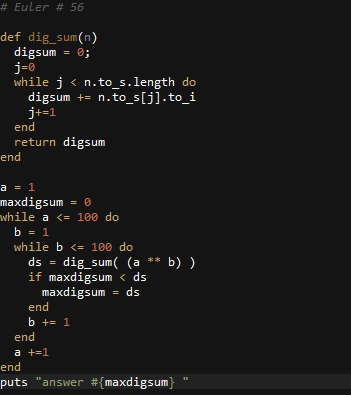# Ruby: Solution to Project Euler Problem # 56

http://projecteuler.net/problem=56

Powerful digit sum

A googol (10100) is a massive number: one followed by one-hundred zeros; 100100 is almost unimaginably large: one followed by two-hundred zeros. Despite their size, the sum of the digits in each number is only 1.

Considering natural numbers of the form, ab, where a, b100, what is the maximum digital sum?